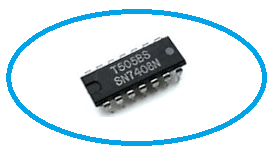## IC 7408

IC 7408 is a logic gate IC. It consists of four two-input AND Gates. The AND gate perform logical AND operation. Logic gates come in form of ICs. The all four AND gates are independent. Each gate has three pins two inputs and one output. IC 74HC08, IC DM7408 are AND gate ICs.

### Pin diagram of IC 7408:

The IC 7408 has total fourteen pins including ground and Vcc. The simple pin diagram is shown below.

#### Operating Condition of IC DM7408 :

• The power supply should be given to the IC from 4.5V DC to 5.25V DC
• The IC can identify a signal as a high-level signal if the voltage of the signal is above 2V
• The IC can identify a signal as a low-level signal if the voltage of the signal is below 0.8V
• The IC should be operated below the 70-degree centigrade

#### Characteristics:

• The IC DM7408 can deliver 21 mA current when the output signal is high at maximum Vcc Voltage.
• The IC can deliver 33 mA current when the output signal is low at maximum Vcc voltage.
• When the Vcc is maximum and the input signal is 5.5V then the IC draws 1 mA current.
• When the Vcc is maximum and the input signal is 2.7V then the IC draws 20 to 40 micro-ampere currents.
• When the Vcc is maximum and the input signal is 0.4V  then the IC draws -1.6 mA current.

### The internal structure of IC 7408:

The internal structure of the IC 7408 is shown it consists of four AND Gates.

### Pin Description of IC 7408:

Pin 1: The pin 1 is the 1st input for 1st AND Gate.

Pin 2: Pin 2 is the 2nd input of 1st AND Gate.

Pin 3: Pin 3 is connected to the output of the 1st AND Gate.

Pin 4: Pin 4 is the 1st input of the 2nd AND Gate.

Pin 5: Pin 5 is connected to the 2nd input of the 2nd AND Gate.

Pin 6: Pin 6 is connected to the output terminal of the 2nd AND Gate.

Pin 7: Pin 7 is the ground pin, it is used to provide power supply to the IC.

Pin 8: It is the output pin of the 3rd AND Gate.

Pin 9: It provides the 2nd input pin for the 3rd Gate.

Pin 10: It is the 1st input pin of the 3rd AND gate

Pin 11: Output of the 4th AND Gate.

Pin 12: It is connected to the 2nd input of the 4th Gate.

Pin 13: The pin 13 is connected to the 1st input of 4th Gate.

Pin 14: It is the Vcc terminal of the IC, it is used to provide the power supply to the IC chip.

### Application of IC 7408:

1.      IC 7408 is used for digital electronics projects.
2.      Used in some electronics devices.

IC 7408 Pin Diagram, Circuit Design, Data Sheet, ApplicationReviewed by manoranjan das on 10:23 am Rating: 5